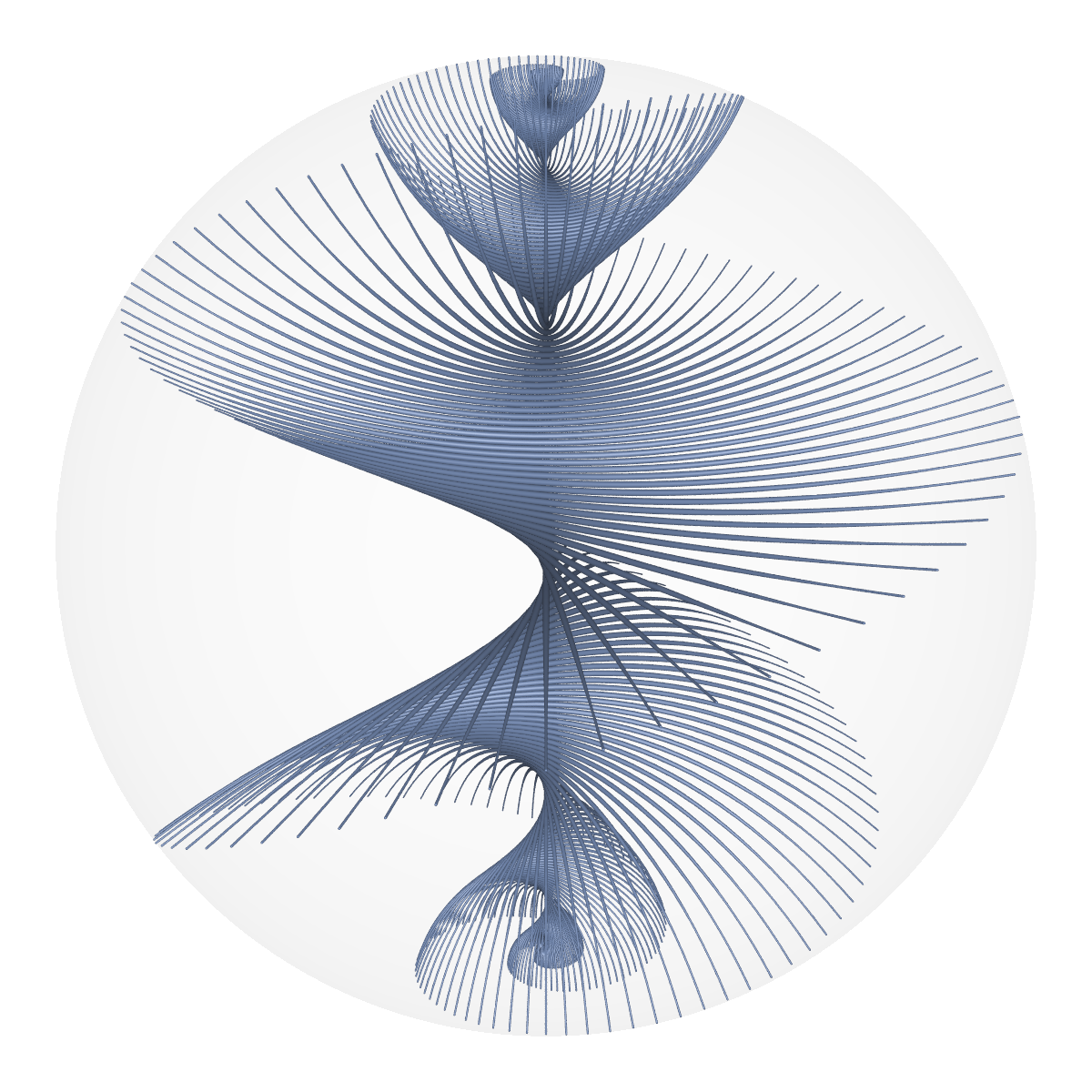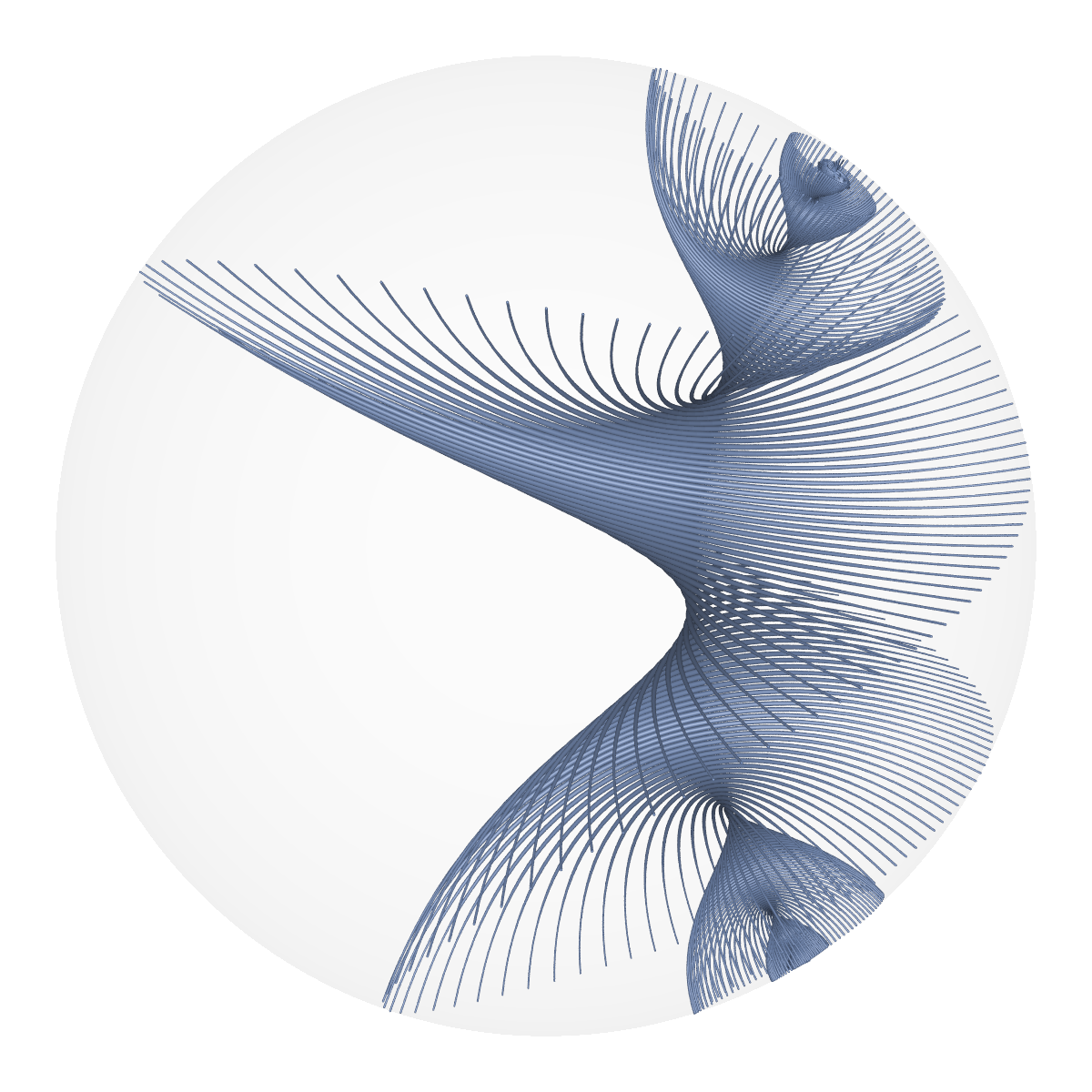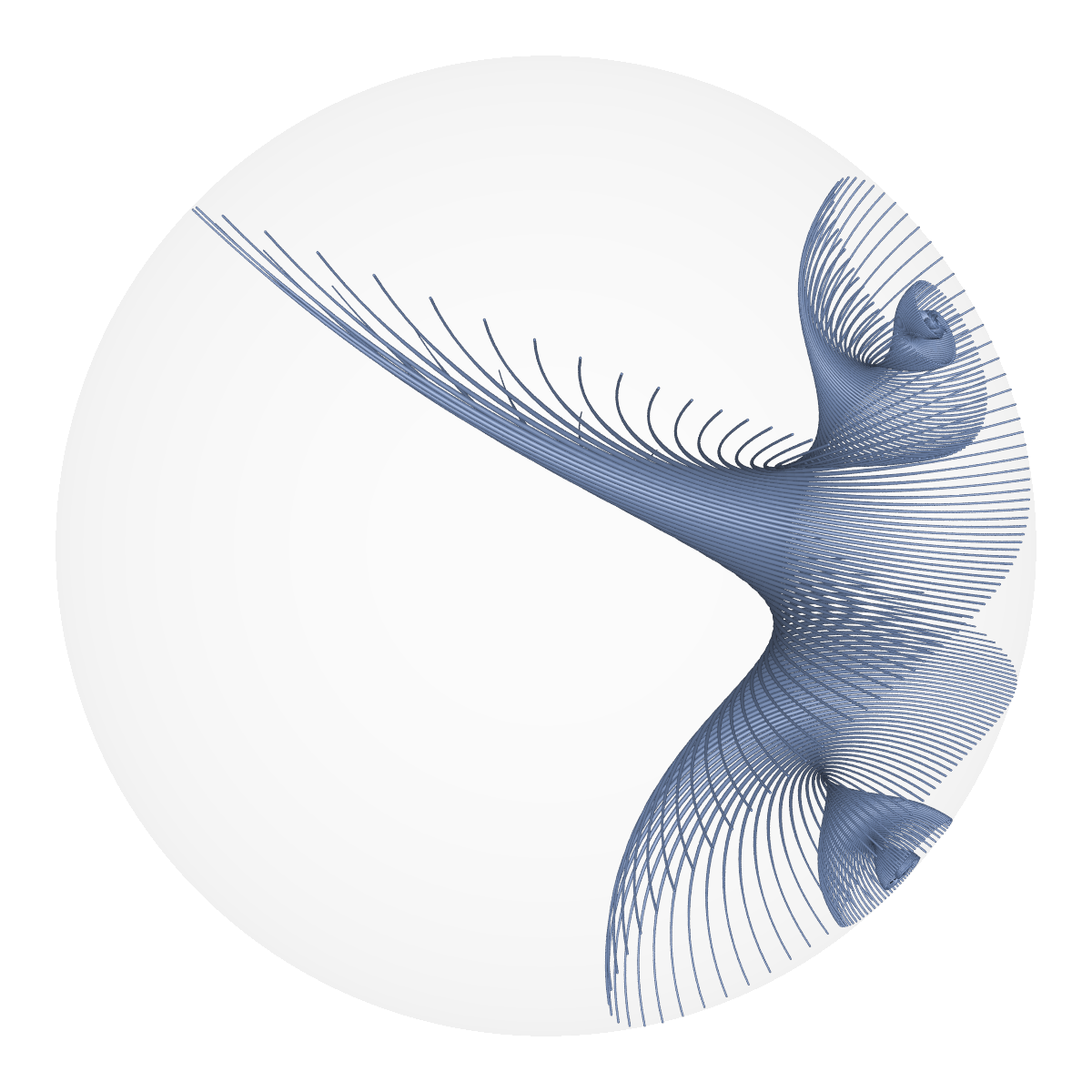### Helicoid in H^3

Helicoid in H^3

Here are three views of a hyperbolic helicoid, an idea suggested by Saul Schleimer. The first puts the helicoid axis on the vertical line through the origin. The other two apply a hyperbolic isometry to move the axis towards the right. Whenever the axis does not go through the origin, it will be an arc in the ball model.

What curve does a single ideal edge of the surface trace out on the ball boundary? I suspect it is a loxodrome, but I haven't proved this. (Update: It is! see comments below.)

Euclidean helicoids
en.wikipedia.org/wiki/Helicoid

Loxodromes
en.wikipedia.org/wiki/Rhumb_line1.Nice! Next: K3 and Calabi-Yau surfaces?

2.Pretty cool! The two boundaries at infinity are definitely loxodromes - this is easier to see in the upper half space model, using polar coordinates on the complex plane at infinity. (Moreover, by conformality, this is also a proof for the ball model.)

3.A loxodrome seems correct to me, because the angle between the helicoid and a plane parallel to the axis should be preserved by the conformal mapping to the sphere at infinity, so you should end up with a line at a constant angle to the meridians of longitude.

4....that is, for the first case, where the mapping to Euclidean space puts the axis at the center.

5.Matt McIrvin, I think if you demonstrate the curve is a loxodrome when the helicoid axis is a line, you can consider the transformed curves loxodromes as well, at least from the perspective of the Mobius transformation formulas for a general loxodrome: roice3.blogspot.com/2008/11/loxodromes.html.

But I see what you mean if you identify loxodromes with rhumb lines, like wikipedia does. I think it'd be nice if wikipedia had a separate article on loxodromes, more focused on them from the perspective of complex analysis.

6.Roice Nelson Yes, all the cases are going to be related by Mobius transformations, so that follows. I was thinking of a loxodrome as a rhumbline.

7.A loxodrome in H3, would be essentially a straight line. Recall that a loxodrome strikes the same angles to perpendiculars to a plane.